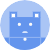search
Search
menu search toc more_vert
Guest 0reps
Thanks for the thanks!
close
chevron_left DataFrame
Basic and Descriptive Statistics29 topics
Sorting and Restructuring DataFrames14 topics
Functions and Aggregations8 topics
Miscellaneous2 topics
Meta Information2 topics
Time Series12 topics
Binary Operators25 topics
Combining DataFrames7 topics
Iterators6 topics
Type Conversion7 topics
Constructor DataFrame
Data Indexing and Masks12 topics
Handling Missing Values4 topics
Properties10 topics
Data Selection and Renaming29 topics
Cancel
Post
account_circle
Profile
exit_to_app
Sign out
help Ask a question
search
keyboard_voice
close
Searching Tips
Search for a recipe:
"Creating a table in MySQL"
Search for an API documentation: "@append"
Search for code: "!dataframe"
Apply a tag filter: "#python"
Useful Shortcuts
/ to open search panel
Esc to close search panel
to navigate between search results
d to clear all current filters
Enter to expand content previewDoc SearchCode Search BetaSORRY NOTHING FOUND!
mic
Start speaking...Voice search is only supported in Safari and Chrome.
Shrink
Navigate to
A
A
brightness_medium
share
arrow_backShareTwitterFacebook
chevron_left DataFrame
Basic and Descriptive Statistics29 topics
Sorting and Restructuring DataFrames14 topics
Functions and Aggregations8 topics
Miscellaneous2 topics
Meta Information2 topics
Time Series12 topics
Binary Operators25 topics
Combining DataFrames7 topics
Iterators6 topics
Type Conversion7 topics
Constructor DataFrame
Data Indexing and Masks12 topics
Handling Missing Values4 topics
Properties10 topics
Data Selection and Renaming29 topics
0
thumb_down
0
chat_bubble_outline
0
auto_stories new
settings

# Pandas | DataFrame constructor

Pandas
chevron_right
Documentation
chevron_right
DataFrame
schedule Jul 1, 2022
Last updated
local_offer PythonPandas
Tags

Pandas' `DataFrame(~)` constructor is used to initialise a new DataFrame.

# Parameters

1. `data` | `scalar` or `2D ndarray` or `iterable` or `dict` or `DataFrame`

The `dict` can contain scalars and array-like objects such as lists, Series and NumPy arrays.

2. `index`link | `Index` or `array-like` | `optional`

The index to use for the DataFrame. By default, if `index` is not passed and `data` provides no index, then integer indices will be used.

3. `columns`link | `Index` or `array-like` | `optional`

The column labels to use for the DataFrame. By default, if `columns` is not passed and `data` provides no column labels, then integer indices will be used.

4. `dtype`link | `dtype` | `optional`

The data type to use for the DataFrame if possible. Only one type is allowed, and no error is thrown if type conversion is unsuccessful. By default, `dtype=None`, that is, the data type is inferred.

5. `copy` | `boolean` | `optional`

This parameter is only relevant if `data` is a `DataFrame` or a `2D ndarray`.

• If `True`, then a new DataFrame is returned. Modifying this returned DataFrame will not affect `data`, and vice versa.

• If `False`, then modifying the returned DataFrame will also mutate the original `data`, and vice versa.

By default, `copy=False`.

# Return value

A `DataFrame` object.

# Examples

## Using a dictionary of arrays

To create a DataFrame using a dictionary of arrays:

``` df = pd.DataFrame({"A":[3,4], "B":[5,6]})df A B0 3 51 4 6 ```

Here, the key-value pair of the dictionary is as follows:

• `key`: column label

• `value`: values of that column

Also, since the `data` does not contain any index (i.e. row labels), the default integer indices are used.

## Using a nested dictionary

To create a DataFrame using a nested dictionary:

``` col_one = {"a":3,"b":4}col_two = {"a":5,"b":6}df = pd.DataFrame({"A":col_one, "B":col_two})df A Ba 3 5b 4 6 ```

Here, we've specified the index in `col_one` and `col_two`.

## Using a Series

To create a DataFrame using a Series:

``` s_one = pd.Series([3,4], index=["a","b"])s_two = pd.Series([5,6], index=["a","b"])df = pd.DataFrame({"A":s_one, "B":s_two})df A Ba 3 5b 4 6 ```

## Using 2D array

We can pass in a 2D list or 2D NumPy array like so:

``` df = pd.DataFrame([[3,4],[5,6]])df 0 10 3 41 5 6 ```

Notice how the default row and column labels are integer indices.

## Using a constant

To initialise a DataFrame using a single constant, we need to specify parameters `columns` and `index` so as to define the shape of the DataFrame:

``` pd.DataFrame(2, index=["a","b"], columns=["A","B","C"]) A B Ca 2 2 2b 2 2 2 ```

## Specifying column labels and index

To explicitly set the column labels and index (i.e. row labels):

``` df = pd.DataFrame([[3,4],[5,6]], columns=["A","B"], index=["a","b"])df A Ba 3 4b 5 6 ```

## Specifying dtype

To set a preference for the type of all columns:

``` df = pd.DataFrame([["3",4],["5",6]], dtype=float)df 0 10 3.0 4.01 5.0 6.0 ```

Notice how `"3"` was casted to a `float`.

Note that no error will be thrown even if the type conversion is unsuccessful. For instance:

``` df = pd.DataFrame([["3@@@",4],["5",6]], dtype=float)df 0 10 3@@@ 4.01 5 6.0 ```

Here, the dtypes of the columns are as follow:

``` df.dtypes 0 object1 float64dtype: object ```
mail